## FPGA-in-the-Loop

FPGA-in-the-loop (FIL) enables you to run a Simulink® simulation that is synchronized with an HDL design running on an Intel® or Xilinx® FPGA board. This link between the simulator and the board enables you to verify HDL implementations directly against Simulink or MATLAB® algorithms. You can apply real-world data and test scenarios from these algorithms to the HDL design on the FPGA.

When simulating Wireless HDL Toolbox™ blocks, you must use a streaming sample interface. Streaming sample data, while required for hardware implementations of communications systems, is time-consuming at the FPGA-in-the-loop interface with Simulink.

You can convert from frames to samples and samples to frames either in Simulink or in MATLAB. Depending on your workflow, you can optimize your FPGA-in-the-loop simulation in one of two ways.

One workflow is a Simulink model that imports framed data from MATLAB. This type of model then uses the Frame To Samples and Samples To Frame blocks to convert the data format. For FPGA-in-the-loop, replace these conversion blocks with FIL Frame To Samples and FIL Samples To Frame blocks.

The other workflow is a Simulink model that imports streaming data from MATLAB. This type of model goes with a MATLAB script that uses the `ltehdlFrameToSamples` and `ltehdlSamplesToFrames` functions. For FPGA-in-the-loop, modify your script and Simulink model so that they pass vectors of data to the FPGA-in-the-loop interface.

When you generate a programming file for a FIL target in Simulink, the tool creates a model to compare the FIL simulation with your Simulink design. For Wireless HDL Toolbox designs, the FIL block in that model replicates the sample-streaming interface and sends one sample at a time to the FPGA. Both these modifications construct vectors that make more efficient use of the interface between the Simulink model and the FPGA board.

The instructions that follow show how to modify FPGA-in-the-loop models for the Verify Turbo Decoder with Streaming Data from MATLAB and Verify Turbo Decoder with Framed Data from MATLAB workflow examples.

### FIL Workflow: Framed Data from MATLAB

#### Autogenerated FIL Model

The generated model, including the FIL block that interfaces with the FPGA board, is shown for a model that converts to streaming samples in Simulink. If each sample is represented by multiple values, then the values are flattened into separate ports for FIL.The blue ToFILSrc subsystem branches the sample-stream input of the HDL Algorithm block to the FromFILSrc subsystem. The blue ToFILSink subsystem branches the sample-stream output of the HDL Algorithm block into the Compare subsystem, where it is compared with the output of the HDL Algorithm_fil block. This setup is slow because the model sends only a single sample, and its associated control signals, in each packet to and from the FPGA board.

#### Modified FIL Model

To improve the communication bandwidth with the FPGA board, modify the autogenerated model. The modified model uses the FIL Frame To Samples and FIL Samples To Frame blocks to send one frame at a time.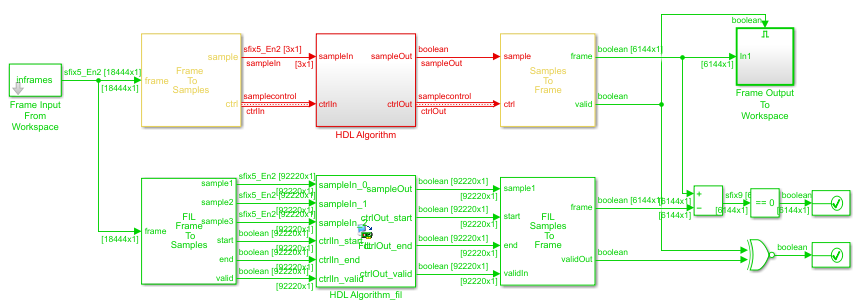To create this modified FIL model:

1. Remove the blue subsystems, and create a branch at the frame input port of the Frame To Samples block.

2. Insert the FIL Frame To Samples block before the HDL Algorithm_fil block. Insert the FIL Samples To Frame block after the HDL Algorithm_fil block.

3. Set the Output frame size on the FIL block to the input frame size.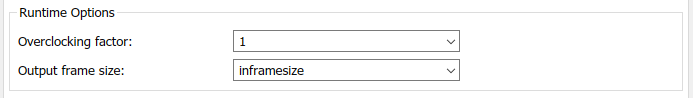4. In the FIL Frame To Samples and FIL Samples To Frame blocks, set the parameters to match the settings of the Frame To Samples and Samples To Frame blocks.

5. Branch the frame output of the Samples To Frame block for comparison. You can compare the entire frame at once with a Diff block. Compare the `validOut` signals using an XOR block.

The input size at the FIL block is the frame size from the input data frames. The vector size of the FIL block ports does not modify the generated HDL code. It affects only the packet size of the communication between the simulator and the FPGA board. This modified model sends an entire frame to the FPGA board in each packet, significantly improving the efficiency of the communication link.

### FIL Workflow: Streaming Data from MATLAB

#### Autogenerated FIL Model

The generated model, including the FIL block that interfaces with the FPGA board, is shown for a model that converts to streaming samples in MATLAB. If each sample is represented by multiple values, then the values are flattened into separate ports for FIL.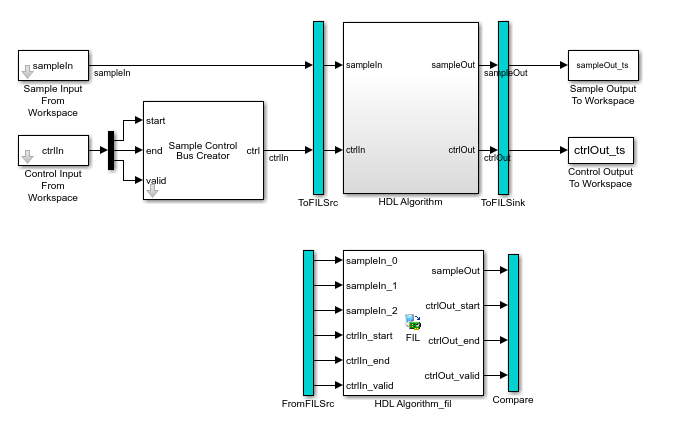The blue ToFILSrc subsystem branches the sample-stream input of the HDL Algorithm block to the FromFILSrc subsystem. The blue ToFILSink subsystem branches the sample-stream output of the HDL Algorithm block into the Compare subsystem, where it is compared with the output of the HDL Algorithm_fil block. This setup is slow because the model sends only a single sample, and its associated control signals, in each packet to and from the FPGA board.

#### Modified FIL Model

To improve the communication bandwidth with the FPGA board, use the generated FIL block in a different model. The alternate model imports and exports vectors of flattened data. The accompanying MATLAB script reshapes the input and output data, and verifies the FIL output against a behavioral model. Reshaping the data in MATLAB is easier and the simulation is faster than reshaping in Simulink.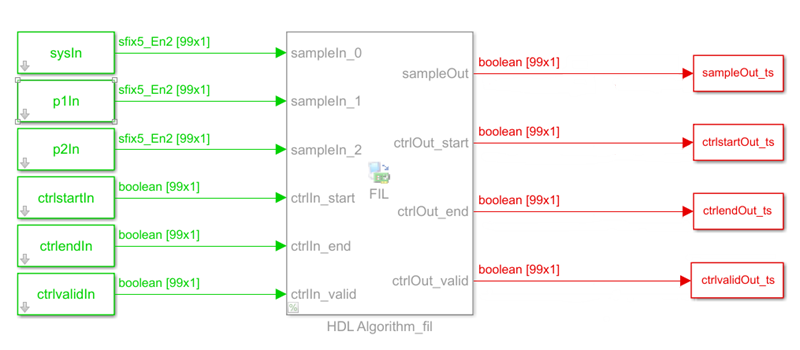First, modify the accompanying MATLAB script:

1. Pick a frame size for the FIL simulation. This size does not have to match the actual frame sizes in the generated data. It can contain your entire data set. The FIL block divides the data into maximum size packets for communication with the FPGA board.

`filframesize = 99;`

2. Combine the cell array of input frames into one matrix.

```allframes = [inframes{:}]; ```

3. Flatten the samples and control signals so there is one vector for each input port on the FIL block. This model includes the LTE Turbo Decoder block, so the input samples consist of three values.

```sysIn = allframes(1:3:end); p1In = allframes(2:3:end); p2In = allframes(3:3:end); ctrlstartIn = ctrlIn(1:3:end); ctrlendIn = ctrlIn(2:3:end); ctrlvalidIn = ctrlIn(3:3:end);```

4. Call the FIL model.

```simTime = size(allframes,1); modelname = 'TurboDecoderStreamingFILVectortoSL'; open_system(modelname); sim(modelname); ```

5. Reshape the output variables for input to the `whdlSamplesToFrames` function. Recreate an N-by-3 control signal matrix and a vector of sample data. In this example, the output sample is a single value. If the output sample is multiple values, build an N-by-SampleSize sample matrix.

```sampleOut = squeeze(sampleOut_ts.Data); ctrlOut = [squeeze(ctrlstartOut_ts.Data) ... squeeze(ctrlendOut_ts.Data) ... squeeze(ctrlvalidOut_ts.Data)];```

1. Copy the generated FIL block into a new model.

2. Configure and connect a Signal From Workspace block for each input port on the FIL block. Use the variables from your MATLAB script as the parameter values.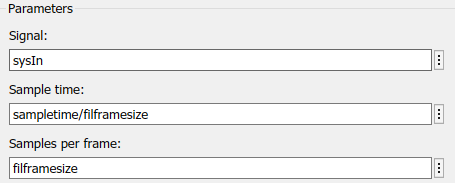3. Set the Output frame size on the FIL block to the desired FIL frame size.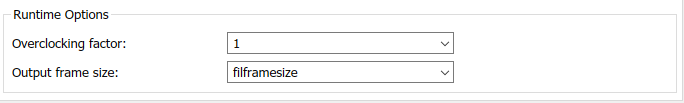4. Configure and connect a To Workspace block for each output port of the FIL block.

The input size at the FIL block is the frame size you specify on the Signal To Workspace blocks. The vector size of the FIL block ports does not modify the generated HDL code. It affects only the packet size of the communication between the simulator and the FPGA board. This modified model sends an entire frame to the FPGA board in each packet, significantly improving the efficiency of the communication link.